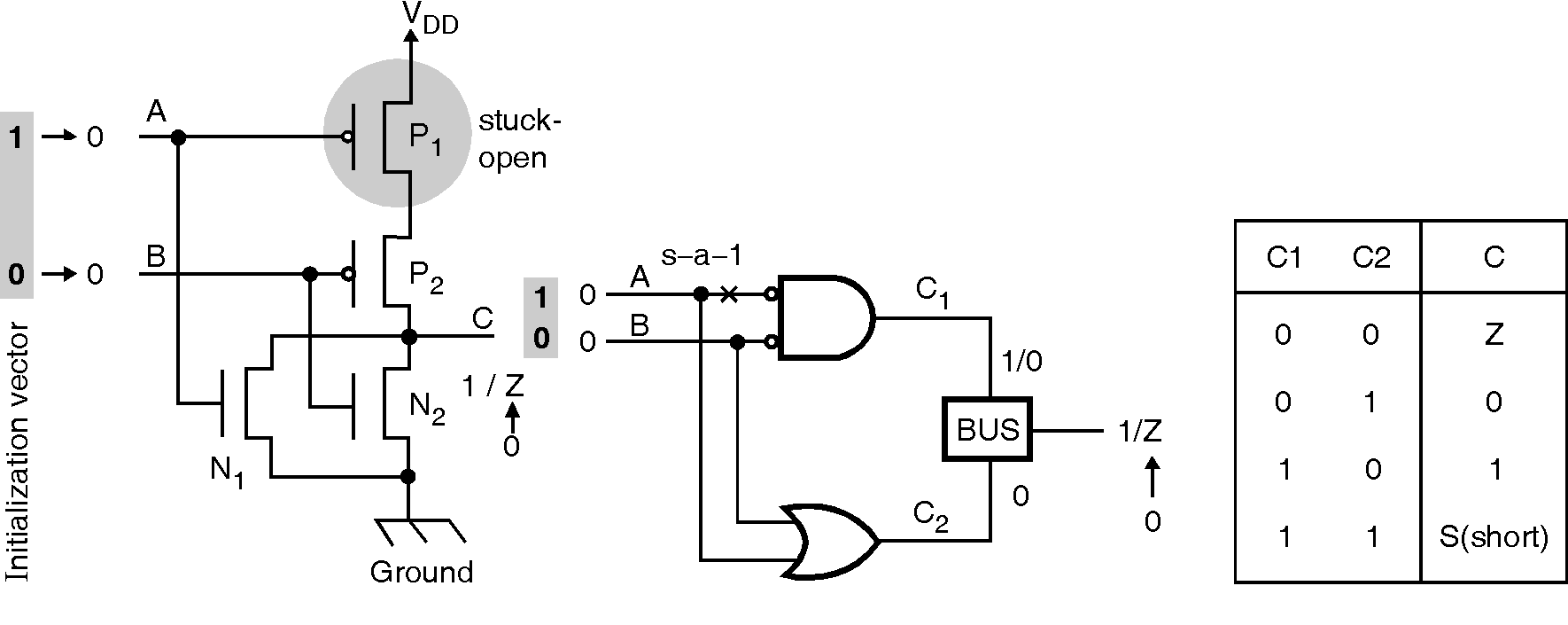﻿ VLSI Design for Testability

Stuck-open and Stuck-short Faults

Stuck-open and stuck-short faults are generally referred to as transistor faults. Faults at the physical level are called defects. The electrical or logic-level faults that can be produced by physical defects are classified as defect-oriented faults. Examples of physical defects are broken wires, bridges, improper semiconductor doping, and improperly formed devices. To understand the operation of purely digital MOS circuits, the simple model of the transistor is useful. A MOS transistor as a switch, a defect is modeled as the switch being permanently in either the open or the shorted state.Stuck-Open Fault :

Figure shows a NOR gate implemented using CMOS technology. P1 and P2 are PMOS transistors when the gate terminal inputs A and B are 0. Further, the inputs A and B also applied at the gate of NMOS transistors, N1 and N2, If A = B = 0 then P1 and P2 are shorted in the fault-free circuit and only P2 is shorted in the faulty circuit. N1 and N2 are open in both circuits. In CMOS circuit output C has some parasitic capacitance with the charge from the previous operation of the circuit. In order to detect the fault, Z assumes value 0.  The test vectors are, 10 ® 00 which produces an output 0 ® 1 in the good circuit and 0 ® 0 in the faulty circuit. Figure also shows gate level model of the CMOS NOR circuit. Here every series interconnection between a supply node to output is replaced by AND gate. Further, a parallel interconnection is replaced by an OR gate. The output is produced by a BUS network whose truth table is shown in Figure. Furthermore, as unknown three-state simulation, the state in this model indicates the short circuit between the supply nodes. Stuck-open fault of a PMOS transistor is modeled as a stuck-at-1 fault at the corresponding input signal and that of an NMOS transistor as a stuck-at-0 fault.

Stuck-Short Fault :

In the gate-level model of above figure a stuck-short fault of a PMOS transistor is represented as a stuck-at-0 fault at the corresponding input to a logic gate. Further, a stuck-short fault of an NMOS transistor is represented as a stuck-at-1 fault of a gate input. For detecting the fault P1 stuck short, a stuck-at-0 fault at the site where stuck-at-1 is shown in the figure. The input vector 10 produces a 0/S output at C. Since S represents a short circuit between the supply nodes, this test produces a high current in the faulty circuit when it reaches the steady state. This current is orders of magnitude larger than the normal quiescent current of a CMOS circuit. Therefore, measurement of device current detects the fault.